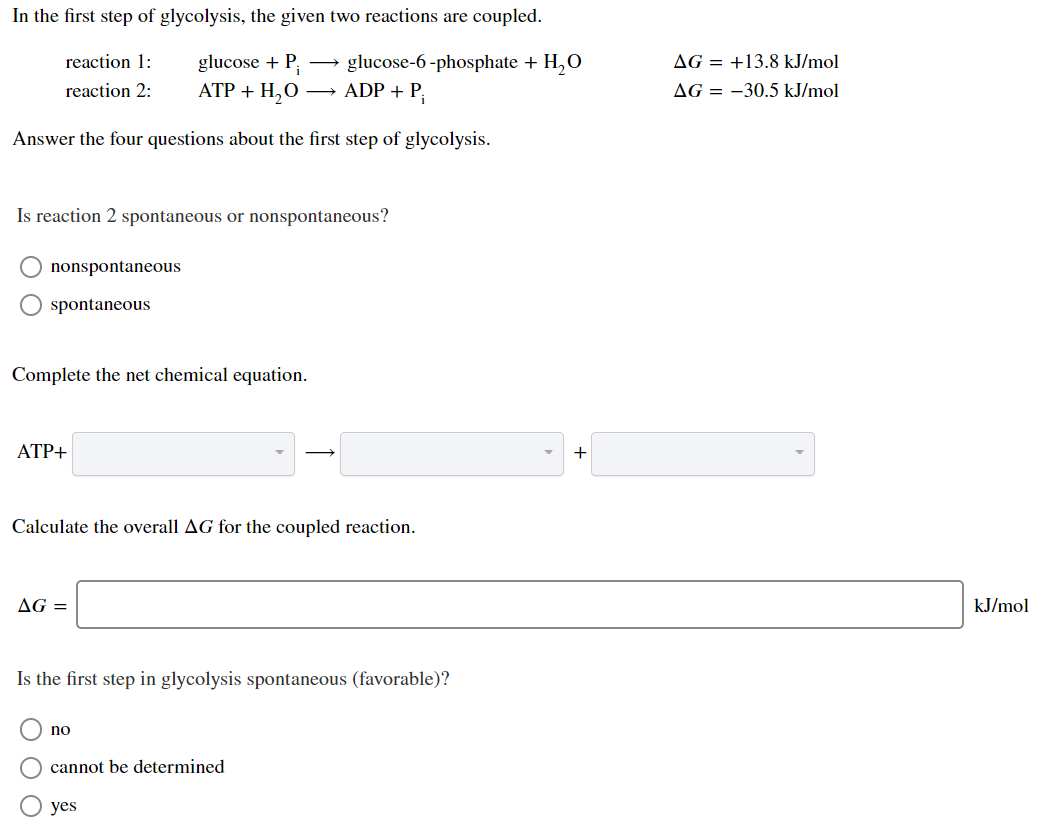# In the first step of glycolysis, the given two reactions are coupled. reaction 1: reaction 2:...

###### Question:In the first step of glycolysis, the given two reactions are coupled. reaction 1: reaction 2: glucose +P: ATP + H2O glucose-6-phosphate + H,O + ADP + P AG = +13.8 kJ/mol AG = -30.5 kJ/mol Answer the four questions about the first step of glycolysis. Is reaction 2 spontaneous or nonspontaneous? O nonspontaneous O spontaneous Complete the net chemical equation. ATP+ + Calculate the overall AG for the coupled reaction. AG = kJ/mol Is the first step in glycolysis spontaneous (favorable)? O no O cannot be determined O yes

##### What is the equation of the line tangent to f(x)= 3x^2 +x-5  at x=-1?
What is the equation of the line tangent to f(x)= 3x^2 +x-5  at x=-1?...